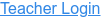### Topics

• Partial Products
• Factors
• Multiplication
• Area Model

### Description

Build rectangles of various sizes and relate multiplication to area. Discover new strategies for multiplying large numbers. Use the game screen to test your problem solving strategies!

### Sample Learning Goals

• Recognize that area represents the product of two numbers and is additive.
• Represent a multiplication problem as the area of a rectangle, proportionally or using generic area.
• Develop and justify a strategy to determine the product of two multi-digit numbers by representing the product as an area or the sum of areas.
Version 1.0.0